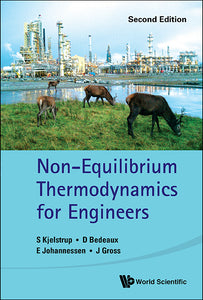# Non-equilibrium Thermodynamics For Engineers (Second Edition)

Signe Kjelstrup

Format: Print Book

ISBN: 9789813200302

• SGD 133.75
Unit price per
Tax included.

Kjelstrup, Bedeaux, Johannessen, and Gross describe what non-equilibrium thermodynamics is in a simple and practical way and how it can add to engineering design. They explain how to describe proper equations of transport that are more precise than those used so far, and how to use them to understand the waste of energy resources in central process units in the industry. The authors introduce the entropy balance as an additional equation to use in engineering; to create consistent thermodynamic models, and to systematically minimize energy losses that are connected with the transport of heat, mass, charge and momentum.Non-equilibrium Thermodynamics for Engineers teaches the essence of non-equilibrium thermodynamics and its applications at a level comprehensible to engineering students, practitioner engineers, and scientists working on industrial problems. The book may be used as a textbook in basic engineering curricula or graduate courses.

Format: Hardcover
No of Pages: 300
Imprint: World Scientific
Publication date: 20170215